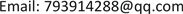1. 引言

2. Eviews中的随机抽样函数

Eviews中提供了18种随机变量的随机抽样函数，都是以@r开头，加上相应的分布名称，如表1所示(括号中是分布参数)    ：

Random sampling function in Eview

Beta分布@rbeta(a,b)Gamma分布@rgamma(r,s)
Lodistic分布@rlogistic(x)帕累托分布@rpareto(a,k)

t分布@rtdist(v)F分布@rfdist(v1,v2)

3. 估计圆周率的随机试验

wfcreate mywork u 1 10000

'建立工作文件，命名为mywork，范围是1~10000，即模拟10000次

series x=@runif(0,1)

'建立序列x，其值是从均匀分布中随机抽取，10000个，也可用rnd函数得到

series y=@runif(0,1)

scat x y

'查看散点图

genr z=4*((x-0.5)^2+(y-0.5)^2<0.5^2)

'生成新序列z，如果点(x,y)落在圆内，则取值为4，否则取值为0

z.stats

'查看序列z的描述性统计量的值

4. 对随机变量的函数分布的验证

wfcreatemyfillu11000

series x=@rnorm

'创建序列x，数值随机抽取于N(0,1)

genr x2=x^2

'生成新序列x2，X2=X2

x2.hist

'绘制序列x2的直方图

series y=1+0.5*@rnorm

'创建序列y，数值来自于N(1,0.52)

genr expy=exp(y)

'生成新序列expy，expy=exp(y)

expy.hist

'绘制序列expy的直方图

5. 对样本统计量的随机模拟

5.1. 对样本均值分布的随机模拟

wfcreatemyworku11000

for !k=1 to 1000

'定义控制变量!k，建立循环，循环1000次

series x{!k}

'建立序列x{!k}

series y{!k}

smpl 1 200

'调整样本范围到1~200

x{!k}=1+2*@rnorm

y{!k}=@rexp(3)

'序列y{!k}的值来自参数是3的指数分布

scalar xm=@mean(x{!k})

'计算序列x{!k}的样本均值

scalar ym=@mean(y{!k})

smpl 1 1000

'恢复工作文件范围到1~1000

matrix(1000,2)mat

'建立矩阵mat(1000´2)

mat(!k,1)=@mean(x{!k})

'将序列x{!k}的均值存放于矩阵mat第一列中

mat(!k,2)=@mean(y{!k})

next

'循环结束

deletex??x???y??y???

'删除一些序列(?是通配符)

mtos(mat,g)

'将矩阵mat转化为两个序列(默认名称是ser01和ser02)

ser01.hist

'查看序列ser01的直方图

ser02.hist

5.2. 对正态分布的样本偏度和峰度分布的随机模拟

wfcreatemyfileu1300

for !k=1 to 2000

series x{!k}

x{!k}=1+2*@rnorm

scalar xs=@skew(x{!k})

'计算序列x{!k}的样本偏度

scalar xk=@kurt(x{!k})

'计算序列x{!k}的样本峰度

matrix(2000,2)mat1

mat1(!k,1)=@skew(x{!k})

mat1(!k,2)=@kurt(x{!k})

next

deletex??x???

range 1 2000

'扩大工作文件范围为1~2000

mtos(mat1,g)

ser01.qqplot

'查看序列ser01的qq图(分位数-分位数图)

ser02.qqplot

5.3. 对相关系数的分布的模拟

wfcreate xishu u 1 10000

series rxy

for !a=1 to 10000

smpl 1 500

series x=@rnorm

series y=@rnorm

scalar r=@cor(x,y)

'计算样本相关系数r

rxy(!a)=r

'将样本相关系数存放在序列rxy中

next

smpl 1 10000

rxy.hist

rxy.distplot cdf

'查看序列rxy的经验分布函数图

6. 对估计量的随机模拟

wfcreate my u 1 2000

series x

x(1)=-10

'序列x的第1个值赋值为-10

smpl 2 21

x=x(-1)+1

'序列x的前一个值加1为下一个值

smpl 1 21

for !k=1 to 2000

series y{!k}

y{!k}=2+0.2*x+3*@rnorm

equation eq{!k}.ls y{!k} c x

'建立方程对象，名称为eq{!k}，用最小二乘法估计

matrix(2000,3)ma

ma({!k},1)=c(1)

ma({!k},2)=c(2)

ma({!k},3)=@se

'矩阵ma的第三列存放每次估计的标准误

next

smpl 1 2000

mtos(ma,g)

freeze(g1) ser01.hist

'冻结ser01的直方图，名称为g1

freeze(g2) ser02.hist

freeze(g3) ser03.hist

graph graph01.merge g1 g2 g3

'合并三个直方图,名称为graph01

graph01.align(3,1,1)

'将三个直方图横向排列

7. 结语